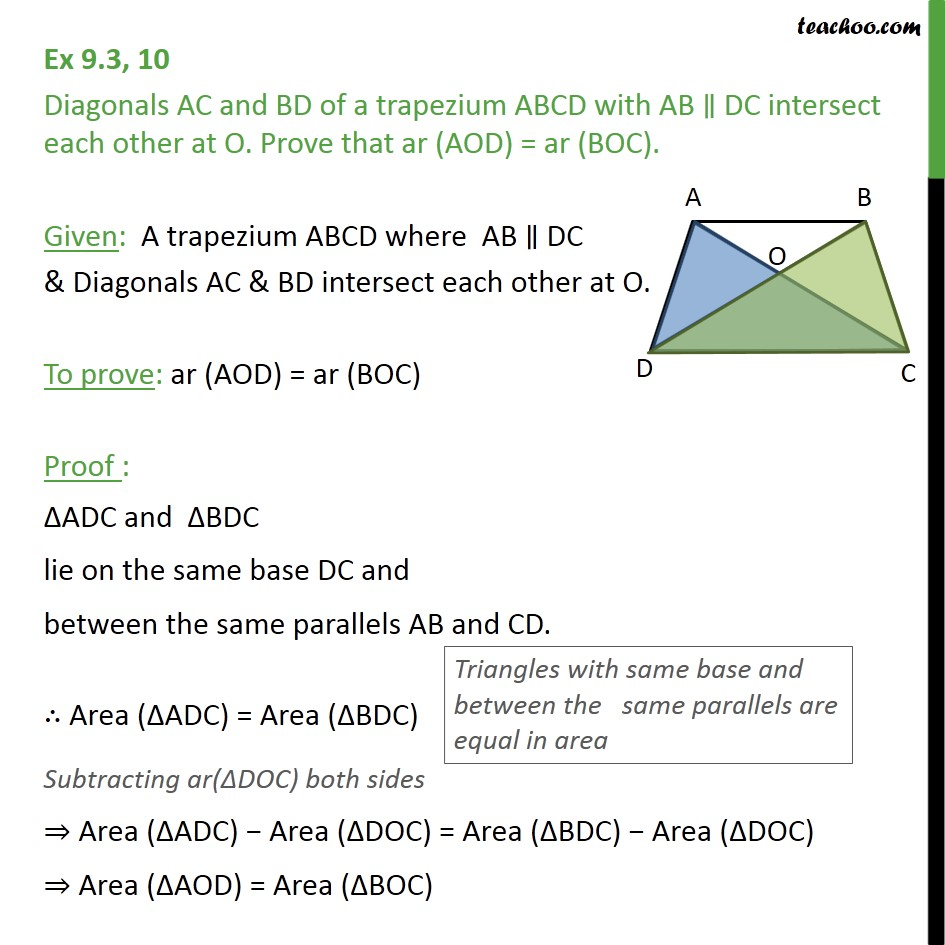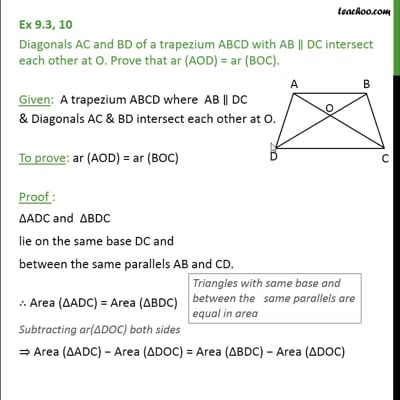Area of Triangles

Areas of Parallelograms and Triangles
Serial order wiseThis video is only available for Teachoo black users

Learn in your speed, with individual attention - Teachoo Maths 1-on-1 Class

### Transcript

Question 10 Diagonals AC and BD of a trapezium ABCD with AB ∥ DC intersect each other at O. Prove that ar (AOD) = ar (BOC). Given: A trapezium ABCD where AB ∥ DC & Diagonals AC & BD intersect each other at O. To prove: ar (AOD) = ar (BOC) Proof : ΔADC and ΔBDC lie on the same base DC and between the same parallels AB and CD. ∴ Area (ΔADC) = Area (ΔBDC) Subtracting ar(ΔDOC) both sides ⇒ Area (ΔADC) − Area (ΔDOC) = Area (ΔBDC) − Area (ΔDOC) ⇒ Area (ΔAOD) = Area (ΔBOC)